# X-Carve Tutorial

There’s a strange machine in the workshop!
It’s big and interesting, but how do you make it do something useful?

This post will answer that, though it won’t make you a master machinist in one go.
I’ll assumes that you already made a design, and now you want to fabricate it.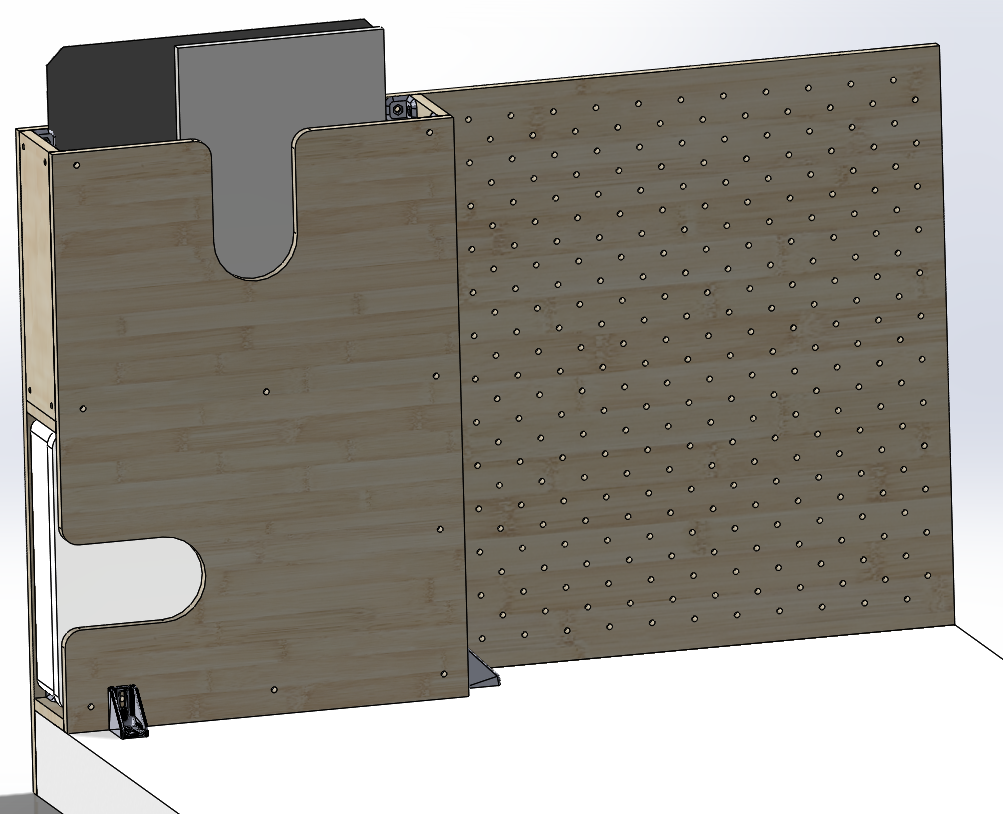Here’s one I prepared earlier…

# Part 0: X-Carve and Easel

The X-Carve comes with some cloud-based software called “Easel”.
There’s been plenty of hate directed at it. Easel is nowhere near perfect, but it can be wrangled into submission, and it can even be useful.

If you have complex requirements you might actually need something more advanced.
It’s possible to side-step Easel entirely, but it is way more complex, and not necessary for 95% of users.
Not typically worth it. So use Easel. Grumble if you want.

Easel works in the browser. Boo. On the plus side;

• You can complete a lot of the work (All of Part A and Part B below) before you even get to the workshop or in front of the X-Carve PC.
• If you login to Easel on the X-Carve PC using an “Incognito” browser tab, then you’ll see only your account, and nobody else will mess with or be confused by your stuff after you close the tab.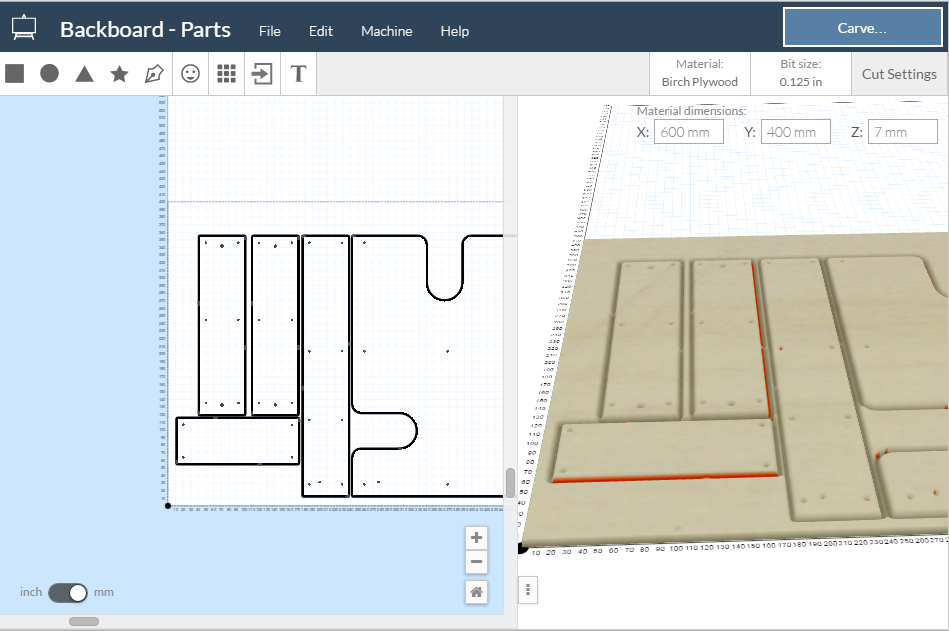“I am Easel!”

# Part A: Import

Easel only supports SVG import, not DXF.
Lots of scaling issues can occur when passing SVG files between programs. Blech.

Here’s a whole section just on getting your design into Easel – I have found a process that works:

1. Get source file.
2. Grumble at Easel for not supporting DXF import yet,
and that SVG is a terrible format for parts with physical dimensions.
3. Start a new Inkscape document.
Import the DXF file. (Do not open the DXF file or the dimensions will be correct in Inkscape but wrong in Easel!)
4. Import any other shapes/files you want to cut, and arrange them how you like.
5. Go to document properties, resize drawing to selection, zero margins.
6. Here’s the magic bit:
This joins only the separated parts of each shape together, and allows Easel to know what “inside” and “outside” means.

1. Select all.
2. Ungroup.
3. Select all.
4. Path > Combine.
5. Choose the ‘Edit Path By Nodes’ tool.6. Drag a selection rectangle around the whole design.
7. Join nodes.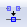8. Leave node editing mode.
9. Select the design. (now a single path)
10. Path > Break apart.
7. Save as an Inkscape SVG.
8. Select all, look up in the toolbar where it says height and width, set view to millimetres. Jot down what it ways the actual dimensions should be.
Think about whether those numbers are realistic!
9. Start a new Easel project.
Import SVG, choose file.
Check that the dimensions match what you expected.
If not, swear. Try to figure out where you went wrong.

Part B: CAM
CAM stands for “computer aided manufacturing”; how to get from a model to instructions that make a physical part.
This step isn’t particularly difficult, but it’s important that you pass the correct instructions to the machine!

## Steps (Summary)

1. Import the SVG file or files.
2. Specify tool, material, dimensions.
3. Choose cutting settings for each path.
4. “Carve…”

## General Tips

• The Easel interface lets you tell it what sort of material you have and its dimensions.
(It also may need reminding about the total bed size – about 800×800 mm)
• A 3D view of the stock and design show up in the preview window, which you can resize smaller to have more room in the working window.

## Path Planning

• Easel assumes that EVERY drawn path will have one cutting operation on it. Selecting the path shows you the options.
• You can choose from;
• Outline on the line, inside, or outside.
• Fill. (Which seeing as you’re doing the opposite is an odd naming choice)
• There’s no specific “drill” operation; if you want a small round hole you draw a small round hole and choose “inside” outline.
• You can specify the depth of the cut:
• By default each cut goes to the full depth of the material.
• You can adjust depth with the vertical slider.
• You can have a path that does not cut at all by setting the depth to zero. (It will turn white) This can be useful when your stock is already the same size as the outline and you just want to cut inside it.
• There’s no specific “drill” operation; if you want a small round hole you draw a small round hole and choose “inside” outline.
• The 1/8″ bit is versatile, and a good choice for many projects.
Plus; chances are it’ll already be mounted in the spindle. Easy!

## Machining Limitations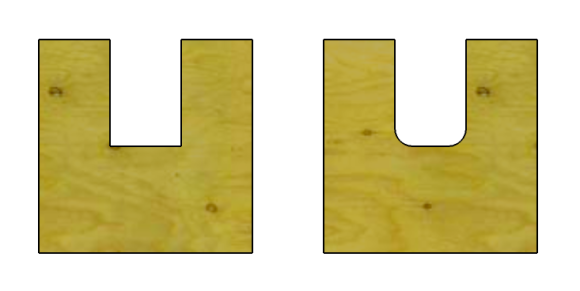• The milling bit can’t reach into corners smaller than its diameter – you may need to file the corners out later, design your thing to have smooth curves, or be fancy and make your own “dog bones“.
(Easel doesn’t know how to do them)
• When cutting holes, the diameter has to be larger than the bit – or Easel will ignore it. Using the 1/8″ bit, the holes should be at least 3.3mm.
(Pressing the “simulate” button will show whether a hole will end up being cut)
• The bed and the material are never perfectly flat. You should overstate the material’s thickness slightly – to ensure that cuts go all the way through.
An extra 0.2 mm is often plenty.
• If a piece is completely cut away, it could jump up and get in the way of the bit. This can be dangerous, noisy and embarrassing, not to mention ruin your piece!
• Easel supports tabs. Tabs are little bits that don’t get cut out, so that the pieces stay put until you cut the last bits by hand.
• There are some small hand-held saws with a hacksaw blade that are perfect for removing tabs – check in the blue tool chest.
• To edit tabs on a path; Click on the path. In the cut menu enable tabs, and choose how many and how large to make them.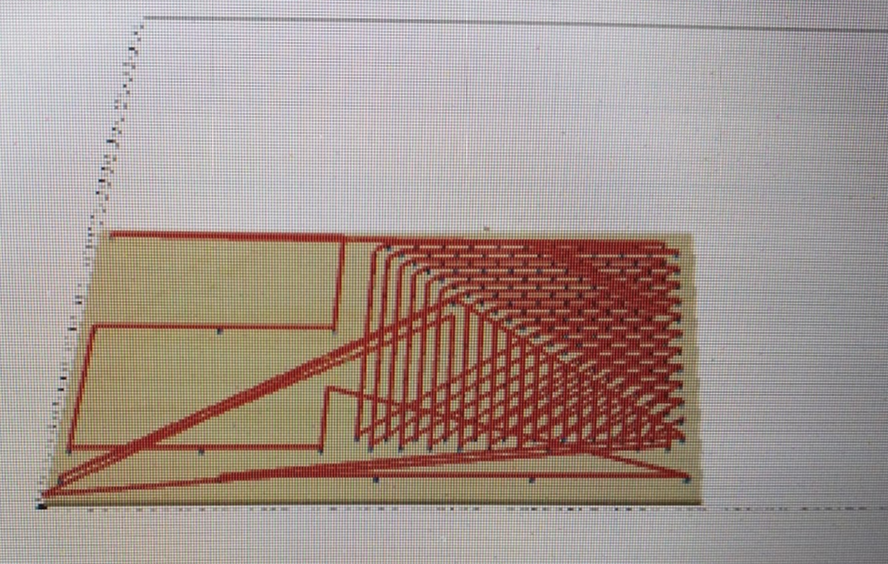This is what >300 holes looks like in ‘simulate’

# Part C: Machining

In order,

1. Spoil Board – Find some material about the same size to go underneath and catch when the bit goes too deep. It’s best if it’s the same material or softer.
Yes, the MDF bed could cope with some abuse, but better to take care of it!
2. Material Alignment – be sure the cuts go where you want them to!
You’ll usually want to align your material so that it’s perpendicular to the board.
One method I like is;

1. Drive two identical screws into the horizontally separated bed holes, leaving them sticking up.
2. Put the spoil board behind them, then pull a straight edge back onto the screws – perpendicular!
3. Place the material on top, and align the edge of the material with the spoil board – now it’s perpendicular too.
3. Clamping – got to stop the material from moving!
This takes some time, be patient and do it properly.

1. Make sure you have plenty of clamping spots.
Especially if the material was a bit warped – it may shift up/down a bit as parts are cut out and mess up your depths or tabs.
2. Make sure the clamps are tightened down well.
If the material moves, the work will be ruined, you’re going to have a bad day, and the machine could be damaged.
3. Make sure the clamps are away from where the cuts will go!
This is important – running into even the wooden part of a clamp might damage the machine or break a bit.
4. Insert Bitskip if already the right size.
1. There are two spanners in the middle drawer.
Place both on the spindle and turn the lower one left, the upper one right.
Carefully loosen until you can get the old bit out.
2. Place the new bit in the spindle, with plenty of shaft inside.
If the shaft is a different diameter too, then the collet (inside the spindle) will need to be changed as well.
3. Tighten back up again until the bit is firmly seated.
5. Head Alignment – set up the head in the right place.
With the machine off (push the big red button) gently pull/push the gantry and the head until the bit is right on the bottom-left corner of your work.

1. If cutting shapes from inside the material, maybe position the head away from the edge. (to avoid the clamps)
If cutting inside an entire piece of material you’ll use, ensure the head is right on the corner of the material.
2. The gantry should be pulled/pushed from the center, so that one side isn’t stressed.
3. The head can be moved up and down by twisting the pulleys on top.
6. Carve… – noise time!
1. Switch the machine back on. (twist the big red button)
2. Check one more time that you’ve got exactly what you want in Easel, then press “Carve…”.
3. You’ll see a series of final checks – go through them one by one.
“Yes, I have clamped down the material…”
4. When asked to start the spindle motor;
1. Click the button on-screen to raise the head, then
2. Flick the spindle switch next to the read button to ‘On’, then
3. Close the lid, then
4. Click the button on-screen to acknowledge that the spindle is now running.
5. Then it will start moving! Hooray!
The initial speed may be alarming, but as long as you have set up the design and stock correctly, it will stop in just the right place to start the first cut.
6. Once started, you can fold the PC screen down and close the drawer.7. Watch – closely, like you’re babysitting a dozen toddlers in a knife shop.
1. The X-Carve is not like a 3D printer or a laser cutter!
If something goes wrong, it can go wrong badly, quickly.
Here’s some interesting reading on just one kind of failure: http://blog.cnccookbook.com/2014/11/19/survive-first-cnc-router-fire/
2. Stand right there, don’t go back to your seat, don’t go to the bathroom.
Cross your legs if you have to.
3. Know where the big red button is.
Ensure you have a hand free to hit it, if you need to.
4. If you have not selected to use tabs on your cuts,
Be especially wary when the machine finishes cutting a piece completely away. It can jump out and get trapped between the bit and the material. I won’t tell you to reach in there and grab it… use tabs?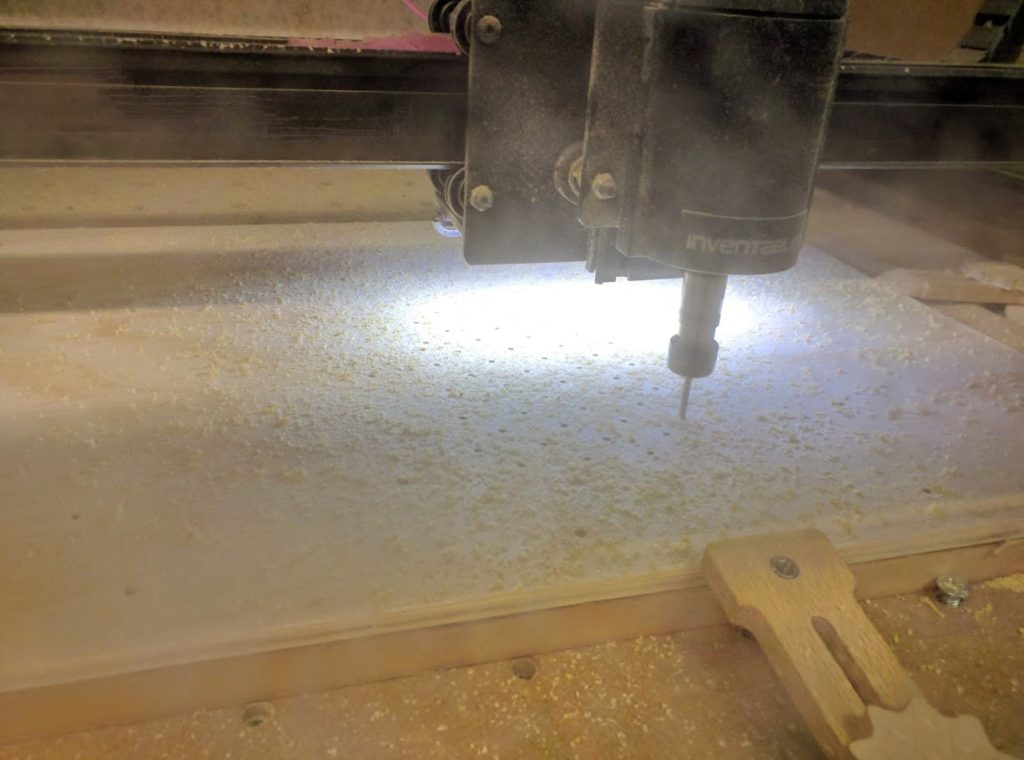8. Cleanup
1. Move the spindle switch to “Off”.
2. Push the big red button to shut down the controller.
3. Now the machine can be considered ‘safe’.
The bit, the spindle motor, or the steppers could still be quite hot.
2. Loosen the clamps.
3. Dust! Everywhere! Shop-vac time.
4. If you used tabs, take a
5. Marvel at your lovely design…

In my previous post, I simulated some mechanical logic gates using a novel 3-bar linkage.
The dimensions of the linkage are critical to achieving correct operation;

Inputs $$\{ false\ false \}$$, $$\{ false\ true \}$$, $$\{ true\ false \}$$ must all produce the same output position.

For the simulations, an empirical approach was used. (fiddle with the values until it looks right)

This time, I’m going to attempt to find the exact values to use for a given stroke, separation distance and output position.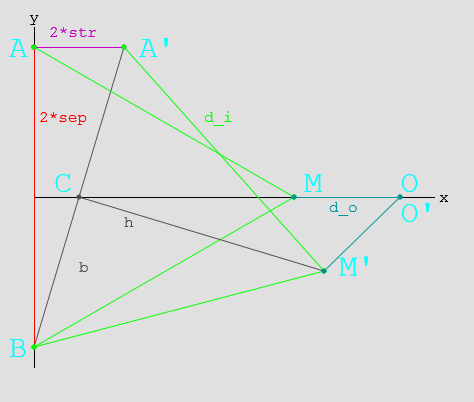Here, you can see the linkage layout for $$\{ false\ false \}$$ and $$\{ true\ false \}$$

# Mathematics

Ahead lurks tough mathematics (which may be unsuitable for liberal-arts majors)
If it’s hard to read, then sorry! It was hard to write!

## Parameters

• $$2 sep$$ Separation between inputs
• $$2 str$$ Stroke length $$false \to true$$
• $$d_i$$ Input link length
• $$d_o$$ Output link length

## Geometry

• $$A(x_a, y_a) = A(0, sep)$$ is the input position when $$A_{state}=false$$
• $$A'(x_a’, y_a’) = A'(2*str, sep)$$ is the input position when $$A_{state}=true$$
• $$B(x_b, y_b) = B(0,-sep)$$ is the input position (The system is symmetric, $$B’$$ does not need to be tested.)
• $$M(x_m, y_m)$$ is the middle position when $$A_{state}=false$$
• $$M'(x_m’, y_m’)$$ is the middle position when $$A_{state}=true$$
• $$O(x_o, y_o)$$ is the output position. ($$O \equiv O’$$ for the gate to function correctly.

## Solving

### Finding $$M$$:

$$M(x_m, y_m) = M( \sqrt{d_i^2 – sep^2}, 0)\\$$

### Finding $$O$$:

\begin{align} O(x_o, y_o) &= O( x_m + d_o, 0 )\\ & = O( \sqrt{d_i^2 – sep^2} + d_o, 0) \end{align}

### Finding $$M’$$:

• $$C'(x_c’, y_c’)$$ is the midpoint of $$BA’$$
• $$b$$ is the length of the line $$BC’$$
• $$h$$ is the length of the line $$C’M’$$
\begin{align} x_c’ &= (x_a’ + x_b)/2 \\ &= str\\ y_c’ &= (y_a’ + y_b)/2 \\ &= 0\\ b &= \sqrt{ sep^2 + str^2 }\\ h &= \sqrt{ d_i^2 – b^2 } && Because BC’ \bot C’M’\\ &= \sqrt{ d_i^2 – sep^2 – str^2 }\\ \end{align}\\

Using this, we can calculate M’:
\begin{align} \frac{h}{b} &= \frac{\sqrt{ d_i^2 – sep^2 – str^2 }}{\sqrt{ sep^2 + str^2 }} \\ &= \sqrt{\frac{ d_i^2 – sep^2 – str^2 }{ sep^2 + str^2 }} \\ &= \sqrt{\frac{d_i^2}{sep^2 + str^2} – 1}\\ \\ x_m’ &= x_c’\ – ( y_c’ – y_b)\frac{h}{b}\\ &= str – \sqrt{\frac{d_i^2}{sep^2 + str^2} – 1} \\ y_m’ &= y_c’ + ( x_c’ – x_b)\frac{h}{b}\\ &= str \sqrt{\frac{d_i^2}{sep^2 + str^2} – 1} \end{align}\\

### Finding $$O’$$:

\begin{align} x_o’ &= x_m’ + \sqrt{ d_o^2 – y_m’^2 }\\ &= ( str – \sqrt{\frac{ d_i^2 }{sep^2 + str^2} – 1}) + \sqrt{ d_o^2 – ( str \sqrt{\frac{d_i^2}{sep^2 + str^2} – 1} )^2 }\\ &= str – \sqrt{\frac{ d_i^2 }{sep^2 + str^2} – 1} + \sqrt{ d_o^2 – str^2 (\frac{d_i^2}{sep^2 + str^2} – 1) }\\ y_o’ &= 0 \end{align} \\

## And then:

Now that $$O$$ and $$O’$$ are found, and we know $$O \equiv O’$$…

### Finding the $$x_o’$$ equation in terms of $$d_o^2$$:

\begin{align} x_o’ &= x_m’ + \sqrt{ d_o^2 – y_m’^2 }\\ d_o^2 – y_m’^2 &= (x_o’ – x_m’)^2 \\ d_o^2 – str^2 (\frac{d_i^2}{sep^2 + str^2} – 1) &= (x_o’ – str + \sqrt{\frac{d_i^2}{sep^2 + str^2} – 1})^2 \\ d_o^2 &= \left( x_o’ – str + \sqrt{\frac{d_i^2}{sep^2 + str^2} – 1} \right)^2 + str^2 (\frac{d_i^2}{sep^2 + str^2} – 1) \\ \end{align}

### Substituting back into the $$x_o$$ equation:

\begin{align} x_o &= \sqrt{d_i^2 – sep^2} + d_o \\ x_o – \sqrt{d_i^2 – sep^2} &= d_o \\ ( x_o – \sqrt{d_i^2 – sep^2} )^2 &= d_o^2 \\ ( x_o – \sqrt{d_i^2 – sep^2} )^2 &= \left( x_o’ – str + \sqrt{\frac{d_i^2}{sep^2 + str^2} – 1} \right)^2 + str^2 (\frac{d_i^2}{sep^2 + str^2} – 1) \end{align}
Ugly, but workable?

# Example:

• $$sep = 50$$ Separation between inputs $$100 mm$$
• $$str = 20$$ Stroke length $$false \to true: 40 mm$$
• $$d_i = ?$$ Input link length
• $$d_o = ?$$ Output link length
• $$x_o = x_o’ = 70$$ Desired output position

\begin{align} ( x_o – \sqrt{d_i^2 – sep^2} )^2 &= \left( x_o’ – str + \sqrt{\frac{d_i^2}{sep^2 + str^2} – 1} \right)^2 + str^2 (\frac{d_i^2}{sep^2 + str^2} – 1) \\ ( 70 – \sqrt{d_i^2 – 50^2} )^2 &= \left( 70 – 20 + \sqrt{\frac{d_i^2}{50^2 + 20^2} – 1} \right)^2 + 20^2 (\frac{d_i^2}{50^2 + 20^2} – 1) \\ \end{align} \\
Pushed through Wolfram Alpha…
\begin{align} \\ d_i &= \sqrt{2900} \\ &= 53.852\\ \text{and}\\ d_o &= x_o – \sqrt{d_i^2 – sep^2} \\ &= 70 – \sqrt{ 2900 – 2500 } \\ &= 50 \end{align}

# Summary:

Phew!

Thusly, one can calculate the input and output linkage lengths for any logic gate.
These dimensions will work for an OR gate too, as the linkages are just reversed.

It’s irksome that I couldn’t work out a symbolic solution for the last equations, especially since $$d_i$$ in the example came out to such a neat value.

I might revisit this further in the future, and it’ll definitely come in handy when building something with these gates.

# Finished: Bubble Wrap Dispenser

This one slipped through the cracks – a Bubble Wrap Dispenser!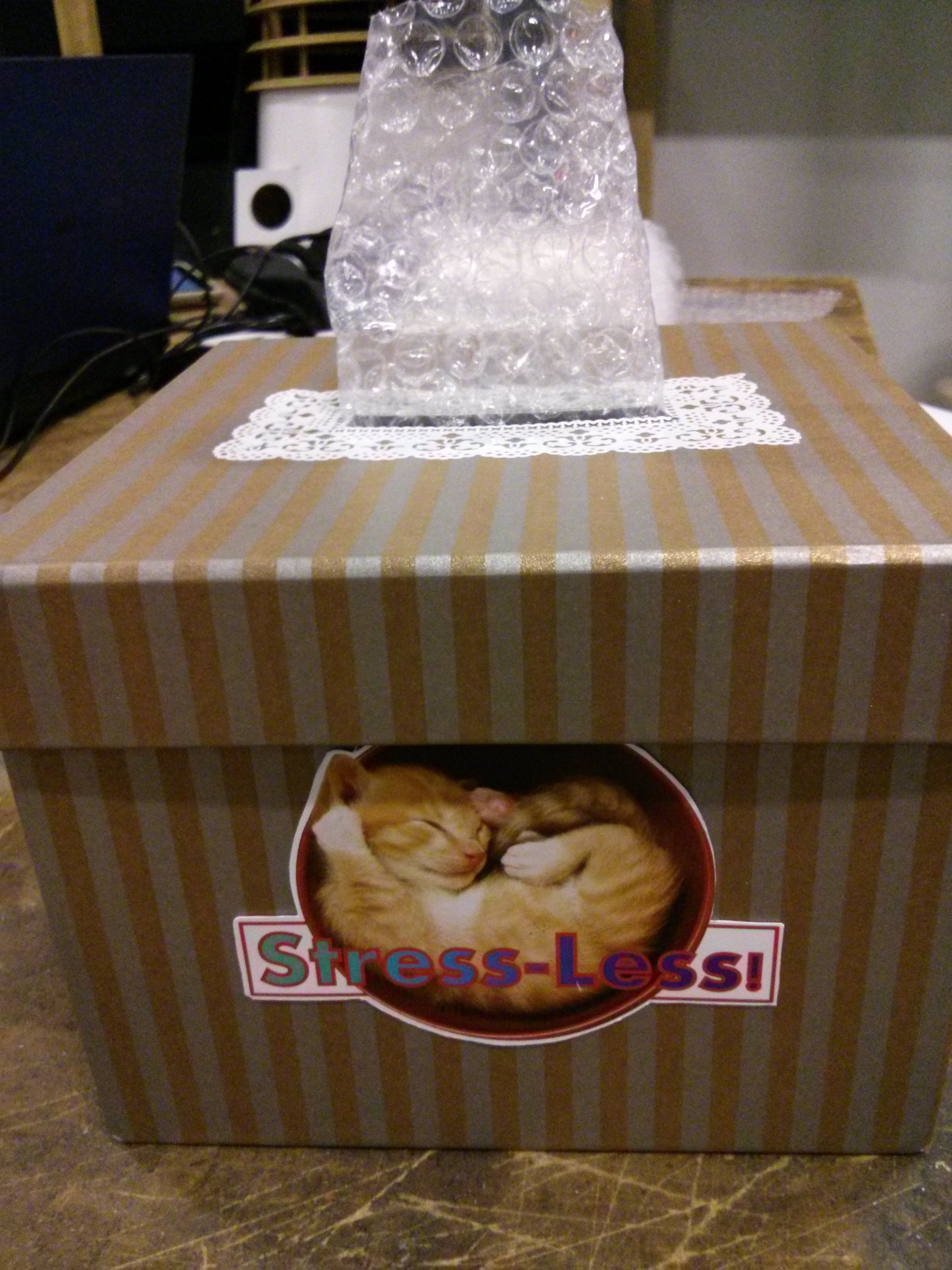Mostly pretty simple to make;

• The box is a gift box from the \$2 shop, with a slot cut in the lid and fancy tape applied.
• The logos are some quick work in Inkscape, printed and cut from glossy paper.
• The bubble wrap…

…was perforated on the laser cutter! I made a dashed line file, and cut the ~5m length of bubble wrap with littls perforations – just pull and tear, and you get a square of bubble wrap.
Worked great, and was really well received. 🙂

# Finished: Garden Box Cat-Proofing

Our cats love munching on greenery. OUR greenery!
This should keep them away, but still allow the sun and rain in.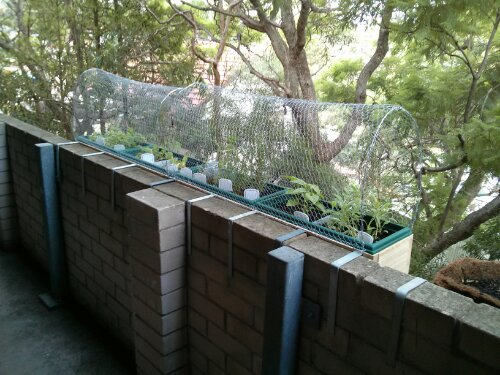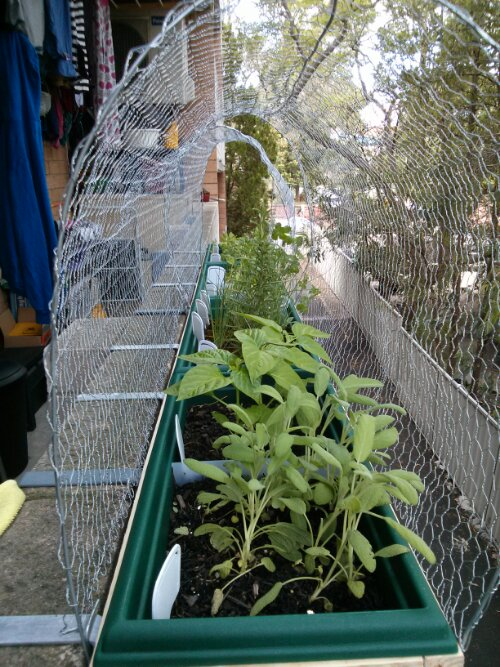Time will tell…

# Ooh, almost finished…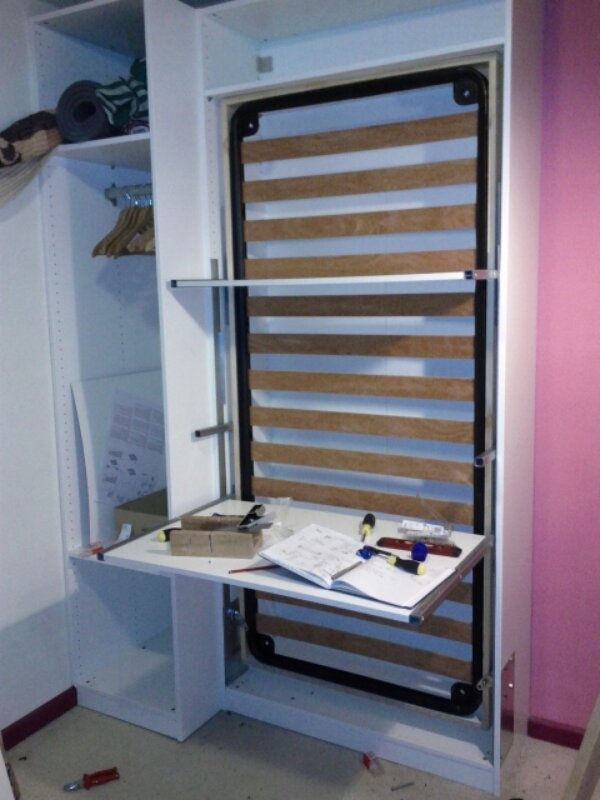Almost completed my DIY folding bed! Most folding beds are just wasted space and volume while folded up. Mine is cleverer than that; it’s a desk and shelf that transforms into a bed. As the bed folds down, the desk and shelf stay level, anything on them is undisturbed.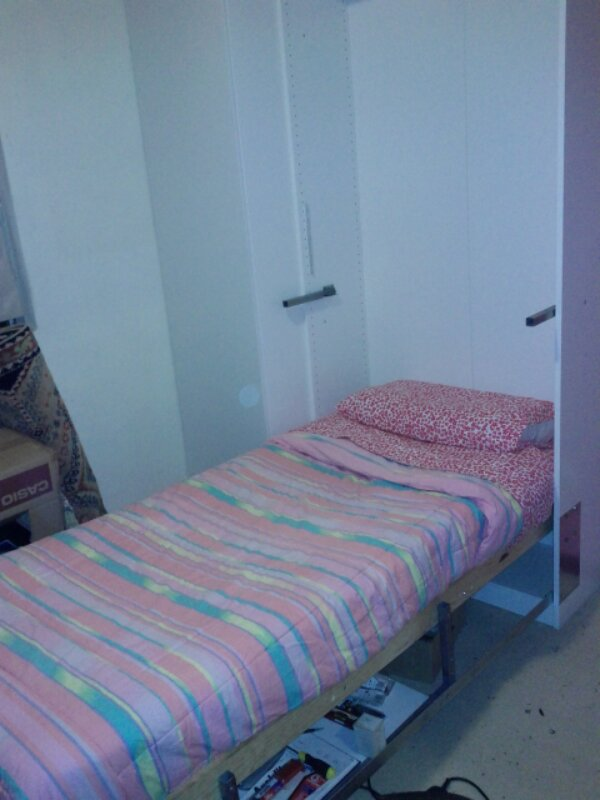# More folding bed linkage design

I’ve refined the earlier linkage design, and managed to simulate it in Phun! (Unfortunately while being easy to use and great for doing simulations, it doesn’t really rate as a proper CAD package; lacking the concept of ‘measurement’ for a start)

Video

Another advantage of this design, I think, is that when the bed is open there’s no gap between the bed and the wall. Many folding beds have this gap, and need a panel anchored to the inner edge of the bed to protect the user rolling down into the mechanism.

A few other challenges have arisen in designing this bed. Namely;

• How to fit a desk somewhere into the design, either that folds down with the bed (so as to end up flat on the floor) or that folds up out of the way before folding the bed down.
• How to fit a wardrobe into the space. The metal bed frame I’m using is 1900 mm tall. The thin IKEA PAX wardrobe is 500 mm wide. The *entire* room is ~2400 mm wide. So maybe I’ll need to figure out how to narrow the wardrobe slightly.
• How to get this all done before my sister-in-law comes to stay!

# Proposed Fold-up Bed Hinge

I need to fit a single bed into the spare bedroom. The best way to do this is probably a wall bed that folds up horizontally, but OUCH they’re expensive!

DIY it is then…

The most complex part is likely to be the hinge mechanism. In a horizontal fold there’s much less work and distance to lift, (Physics?) so I think I can get away with not needing shocks or springs to support the bed.

Another problem is that most folding beds are hinged at the unfolded bed’s height, so that the foldout action clears the rear of the unit. This raises the frame up by ~30cm, making the height of the unit much higher than the mattress width.

I’d love to be able to make some fancy 4-point linkage hinge, but that’s a little tough.

Instead, I think this hinge might work: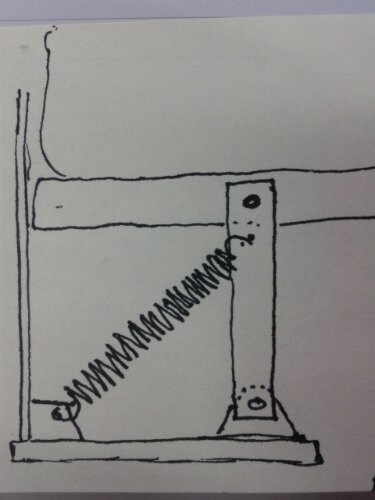This is a side view of one of the two hinges, when the bed is opened. The wall side of the bed rests on the vertical strut shown, which pivots on the bed frame and the base. The spring allows the strut to hinge outward enough to let the mattress transition between horizontal and vertical, but pulls the strut back to vertical when the bed is fully open or closed. (It can’t go further ‘left’ as the bed frame runs up against the back of the unit.)
The outer side of the bed rests on its own braced legs.

What do you think? Good idea? Terrible?

Posted in DIY

# DIY Washing Line

The washing line on my balcony was causing problems; it’s too high for MrsTrick to reach, some parts can’t be used because of the door or the bikes, and it sags when there are heavy things on it. (The span of the balcony is close to 7m)

So I built a better one. 🙂

Went from this: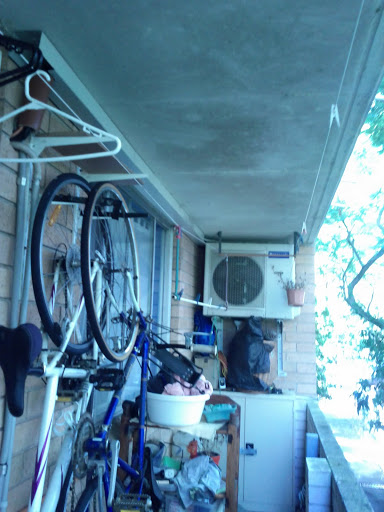To this: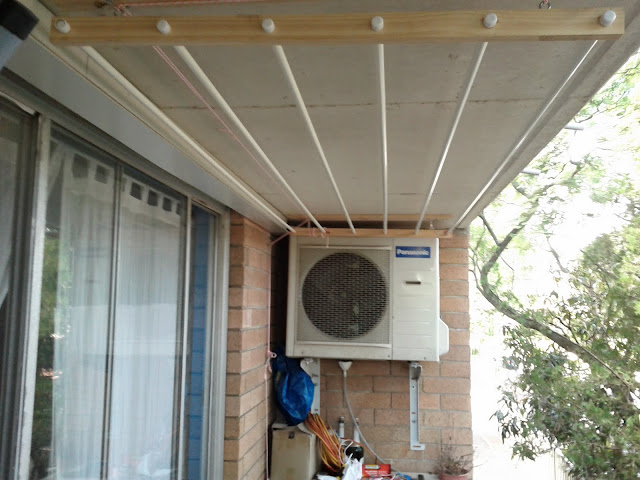• It’s got a simple pulley system so it can be raised or lowered as necessary.
• The rigid curtain rods stop anything from sagging or needing to be retensioned.
• Total hanging space goes from ~12m to ~15m.
• When ‘up’, the clothes are out of the way.
• Assisted drying! (Look at that AC compressor)

Pretty happy with how it turned out. 🙂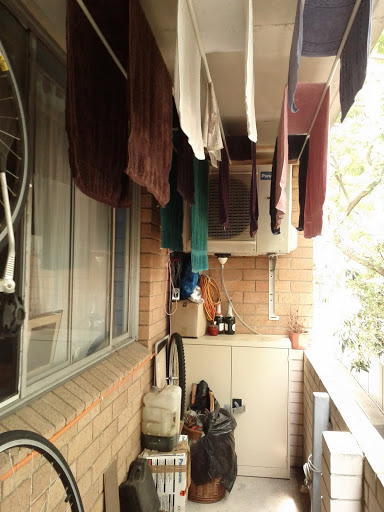More pictures

Posted in DIY